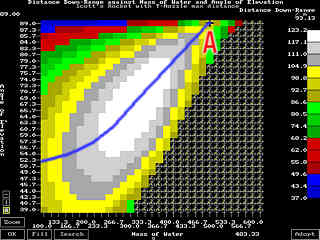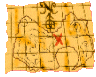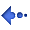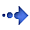Water Rocket Computer Model ProblemsI asked for an explanation for the following . . . 1. to find an explanation for the fact that the amount of water required for a maximum increases with angle of elevation; 2. to find an explanation for the fact that even at an angle of elevation of 89°, the downrange distance is over 93 metres; and, 3. to find an explanation for the fact that the maximum downrange distance is not at an angle of 38° (as in a golf ball) or 45° (as in an artilary shell) or somewhere near that angle.

Again, this rocket uses a Launch Tube and a T-Nozzle. During flight, the rocket slows down slightly, this effect becoming more pronounced as the weight of water increases. As the rocket launches increase in weight, there comes a point where the rocket slows down considerably and starts to tilt over. When this happens at a great enough altitude, the rocket can acheive quite a good downrange distance.

By the time the weight of water and angle of launch have increased as at point A, the rocket almost comes to a stop but a quite a good altitude (answer to question 2). This gradual transition from straightforward flight starting at a low angle, to almost vertical flight, coming almost to a halt in mid-air and then accelerating downrange provides an explanation for question 1.

The reason why the optimum angle is not 38° (as in a golf ball) or 45° (as in an artilary shell) is that the optimum angle depends upon other factors such as the weight of the rocket, the T-Nozzle diameter, the pressure and so on. The optimum angle will vary according to these other variables.Back to the Index Copyright 1994 - 2003 P.A.Grosse. All Rights Reserved